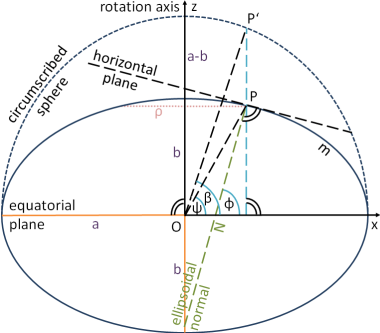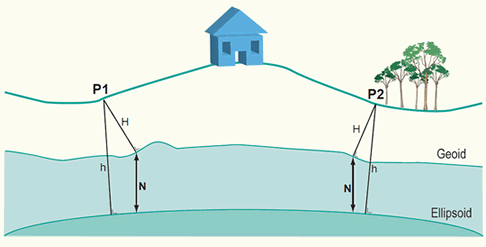A terrestrial reference system (TRS) is a reference system co-rotating with the Earth in its diurnal motion in space. In such a system, coordinates of points attached to the solid surface of the Earth are time-invariant, neglecting geophysical effects such as tectonic or tidal deformations.

Depending on its origin and orientation, a TRS may be geocentric, aka Earth-Centered Earth-Fixed (ECEF); or relative to a point on the Earth's surface, its zenith and meridian: either as local East-North-Up (ENU) or local North-East-Down (NED). TRS coordinates may be Cartesian or geodetic.

Several organizations have designated spatial reference system identifiers (SRID) to uniquely identify commonly used terrestrial reference systems and derived coordinate systems (see Map Projections) for spatial referencing by coordinates (OGC 08-015r2):

• EPSG (European Petroleum Survey Group, now International Association of Oil and Gas Producers) defines over 4000 coordinate reference systems in their EPSG Geodetic Parameter Set; epsg:4326 refers to WGS 84.
• ESRI also defined over 400 SRSs.
• SR-ORG: spatialreference.org records almost 2000 user-defined SRSs.
• IAU2000: International Astronomical Union's (IAU) 2000 Resolutions on Reference Systems defined over 2000 SRSs for several celestial bodies including the Earth.

## International Terrestrial Reference System

The international terrestrial reference system (ITRS) is assimilated to the Geocentric Terrestrial Reference System (GTRS), historically the Conventional Terrestrial Reference System (CTRS), except for a slight difference in time evolution: {IERS Conventions, Chapter 4 Terrestrial reference systems and frames, 2010.}

1. Origin: the geocenter, defined as the Earth's centre of mass including oceans and atmosphere.
2. Orientation axes: Cartesian axes which coincide with Bureau International de l’Heure (BIH) orientation at the epoch 1984.0.
• Reference pole: the Conventional Terrestrial Pole (CTP), an average of the poles from 1900 to 1905.
• Reference meridian: IERS/International Reference Meridian (IRM) passes about 5.3 arcseconds east of the Royal Observatory at Greenwich.
3. Scale: meter, consistent with TCG (Geocentric Coordinate Time).
4. Time evolution: no-net-rotation (NNR) with regards to the horizontal tectonic motions over the whole Earth.

## Geodetic Coordinate Systems

A geodetic coordinate system (GCS, 大地坐标系) is a coordinate system consisting of an spheroid (ellipsoid of revolution) and a meridional plane through the polar axis, or equivalently a geodetic datum.

### Geodetic Datum

Datum (基准面) is a reference or basis for measuring. Geodetic datum (大地基准面), often use interchangeably with datum, is a coordinate system along with a reference ellipsoid that approximates the Earth's surface, specified by at least eight parameters: origin (3) and orientation (3) of the coordinate system, and dimensions (2) of the reference ellipsoid.

World Geodetic System 1984* (WGS 84; EPSG:4326; PROJ.4 +proj=longlat +ellps=WGS84 +datum=WGS84 +no_defs) is the global geodetic datum of the U.S. Department of Defense and the Global Positioning System (GPS): {EUROCONTROL and IfEN, 1998. WGS 84 Implementation Manual.} 1. Coordinate system (aligns with ITRS):

1. Origin (3): Earth's center of mass (error less than 2 cm);
2. Pole (2): Conventional Terrestrial Pole (CTP) for polar motion, as defined by the Bureau International de l'Heure (BIH);
3. Prime meridian (1): the International Reference Meridian, as defined by BIH;
4. Angular velocity: ω = 7.292115 x 10-5 rad/s;
1. Ellipsoid earth model (2):
5. Semi-major axis: a = 6,378,137 m;
6. Inverse flattening: 1/f = 298.257223563;
1. Earth Gravity Field Model:
7. Geocentric gravitational constant including earth’s atmosphere: GM = 398600.5 km3 s-2;
8. Normalized 2nd degree zonal harmonic coefficient of the gravitational potential: c̅_{20} = - 484.16685 x 10-6;
9. Secondary parameters (see reference for details);

Besides WGS 84, geodetic datums with better local fit may be used in different regions, such as North American Datum of 1983 (NAD83) in North America, European Datum 1950 (ED50) and European Terrestrial Reference System 1989 (ETRS89) in Europe, and Ordnance Survey Great Britain 1936 (OSGB36). The WGS 84 datum is almost identical to the NAD83 datum and the ETRS89 datum.

North American Datum of 1927 (NAD27) is the first geodetic datum adopted for the United States. NAD27 is referenced to the Meade’s Ranch Triangulation Station in Kansas (39°13’26.686″N, 98°32’30.506″W), which is near the center of the contiguous United States (CONUS); while NAD83 is geocentric, offset by about 2 meters. NAD27 is uses the Clarke Ellipsoid of 1866: a = 6,378,206.4 m, 1/f = 294.98 (b = 6,356,583.8 m); while NAD83 uses the Geodetic Reference System (GRS80) Ellipsoid: a = 6,378,137.0 m, 1/f = 298.26 (b = 6,356,752.3 m). NAD27 and NAD83 may differ up to 100 meters in horizontal accuracy in CONUS, see NAD27/83 datum shift. You may use NGS Coordinate Conversion and Transformation Tool for general datum shift query.

### Geodetic Coordinates

The coordinates of a point in a geodetic coordinate system are longitude, latitude and altitude/elevation (λ, φ, h):

• (Geodetic) Longitude, λ: the azimuth (方位角) from the meridional plane, with range (−180°, +180°].
• Geodetic Latitude, φ: the complementary angle of the polar angle of an ellipsoidal normal, with range [−90°, +90°].
• Geodetic Height, h: the perpendicular distance of the point from the spheroid.

Geodetic latitude are often confused with two other concepts of latitude. Parametric latitude (β), sometimes called reduced latitude, is the point's parametric angle (离心角) on the meridional ellipse. Parametric latitude is related to the geodetic latitude with $\tan \beta = b/a \tan \varphi$, where a and b are the semimajor and semiminor axes of the ellipsoid. Geocentric latitude, ψ, is the angle from the center of the meridional ellipse. There's no difference between geodetic longitude and geocentric longitude.Figure: (1) Three Latitudes: Geodetic ϕ, Geocentric ψ, and Parametric β. By Rüdiger Lehmann. (2) Deflection of the vertical: astrogeodetic and gravimetric. Adapted from Rishiyur via WikiMedia.

Geodetic longitude and latitude may be referred to as geographic coordinates (地理坐标), which are often expressed in 60-based numbers: an arcminute (arcmin, 角分, ′) is 1/60 of a degree; an arcsecond (arcsec, 角秒, ″) is 1/3600 of a degree. An arcsecond latitude (north/south) is approximately 30.87 meters (2500/81 m, -1.68‰), which remains nearly constant due to the small flattening of the spheroid datum.

In comparison, the astronomic coordinates (天文坐标) for a point on the Earth's surface are the unique coordinates of the direction of the zenith at that point (天顶; opposite to the plumb line): the astronomic longitude is the angle to a selected celestial meridian; the astronomic latitude is the angle to the celestial Equator (天球赤道). Alternatively, the astronomic coordinates may be determined for the zenith orthogonal to the geoid, from the point vertically below. Difference between these two definitions may be significant.Figure: Elevation, H; Geodetic height, h; and Geoidal height, N. Geoid undulation is h-H.

Elevation (height, level; 海拔) is the distance of a point above a specified surface of constant potential, typically the geoid or its approximation, measured along the direction of gravity. As the true elevation is often impossible or extremely difficult to determine, most elevations are approximates, using mean sea level for example, which can differ from the geoid by up to a meter. Geodetic height (大地高), often used as height, is the distance between a point and a geodetic datum, measured along a perpendicular. GPS typically gives geodetic heights. Geoidal height (大地水准面高) is the geodetic height of a point on the geoid. Different from geoidal height, geoid undulation is the difference between elevation and geodetic height. Altitude is a generic term that cannot be technically defined, which casually means the distance of a location above a reference surface along a perpendicular, such as sea level or the physical surface of the Earth.

### Control Datums

Established practice defines a location by combining its horizontal coordinates in one coordinate reference system and its vertical coordinate from another. A control station is a point on the ground whose horizontal or vertical location is used as a basis for obtaining locations of other points. By the kind of coordinates involved, control stations can be classified as horizontal or vertical control. Control datum refers to the datum used to specify locations of control stations.

Types of control datums:

1. Horizontal control datum: geodetic datums are used to define (the horizontal location of) horizontal control points and the associated coordinate systems.
2. Vertical control datum : a set of fundamental (reference) elevations; or the coordinate system specified by them.
• The geoid (大地水准面) is the equipotential surface of the Earth that would coincide with the ocean surface (and hypothetical canals inland) if the oceans and atmosphere were in equilibrium and at rest relative to the rotating Earth; North American Vertical Datum of 1988 (NAVD88) is a vertical control datum based on geoid.
• Tidal datum (潮位基准面) is a curved level surface defined by a certain phase of the tide measured by a tide gauge (验潮仪).
• Sea level datums (海平基准面) are based on mean sea levels (MSL) at specific points over a historic period of time (typically a specific cycle of 18.6 years): Ordnance Datum Newlyn (ODN), the national vertical datum of Great Britain.
• Chart datum (海图基准面) is a datum to which depths in a hydrographic survey or on a nautical chart are referred; typically uses Lowest Astronomical Tide (LAT), the lowest tide predictable from the effects of gravity, or Mean Lower Low Water (MLLW), the average lowest tide of each day.
• Bridge clearances are typically relative to datums based on high tide, such as Highest Astronomical Tide or Mean High Water Springs.

The difference in coordinates between datums is commonly referred to as datum shift.